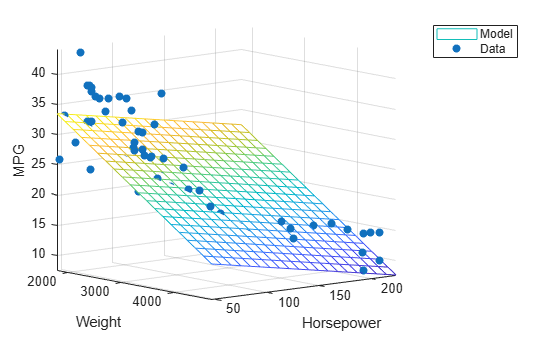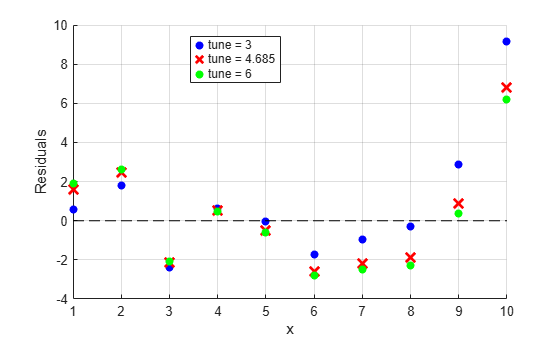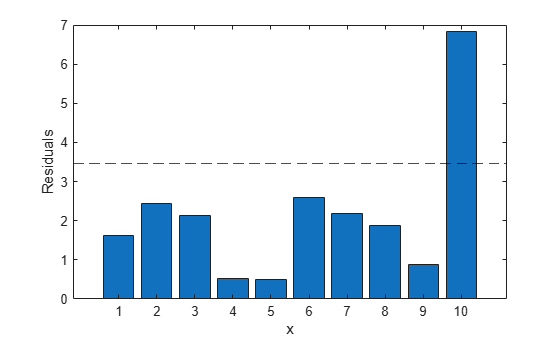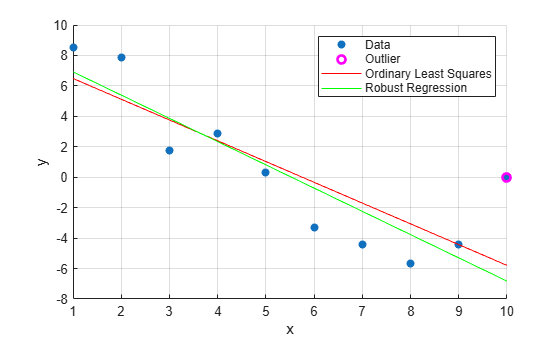# robustfit

Fit robust linear regression

## Syntax

``b = robustfit(X,y)``
``b = robustfit(X,y,wfun,tune,const)``
``[b,stats] = robustfit(___)``

## Description

example

````b = robustfit(X,y)` returns a vector `b` of coefficient estimates for a robust multiple linear regression of the responses in vector `y` on the predictors in matrix `X`.```

example

````b = robustfit(X,y,wfun,tune,const)` specifies the fitting weight function options `wfun` and `tune`, and the indicator `const`, which determines if the model includes a constant term. You can pass in `[]` for `wfun`, `tune`, and `const` to use their default values.```

example

````[b,stats] = robustfit(___)` also returns a structure `stats` containing estimated statistics, using any of the input argument combinations in previous syntaxes.```

## Examples

collapse all

Estimate robust regression coefficients for a multiple linear model.

Load the `carsmall` data set. Specify car weight and horsepower as predictors and mileage per gallon as the response.

```load carsmall x1 = Weight; x2 = Horsepower; X = [x1 x2]; y = MPG;```

Compute the robust regression coefficients.

`b = robustfit(X,y)`
```b = 3×1 47.1975 -0.0068 -0.0333 ```

Plot the fitted model.

```x1fit = linspace(min(x1),max(x1),20); x2fit = linspace(min(x2),max(x2),20); [X1FIT,X2FIT] = meshgrid(x1fit,x2fit); YFIT = b(1) + b(2)*X1FIT + b(3)*X2FIT; mesh(X1FIT,X2FIT,YFIT)```

Plot the data.

```hold on scatter3(x1,x2,y,'filled') hold off xlabel('Weight') ylabel('Horsepower') zlabel('MPG') legend('Model','Data') view(50,10) axis tight```Tune the weight function for robust regression by using different tuning constants.

Generate data with the trend $y=10-2x$, and then change one value to simulate an outlier.

```x = (1:10)'; rng ('default') % For reproducibility y = 10 - 2*x + randn(10,1); y(10) = 0;```

Compute the robust regression residuals using the bisquare weight function for three different tuning constants. The default tuning constant is 4.685.

```tune_const = [3 4.685 6]; for i = 1:length(tune_const) [~,stats] = robustfit(x,y,'bisquare',tune_const(i)); resids(:,i) = stats.resid; end```

Create a plot of the residuals.

```scatter(x,resids(:,1),'b','filled') hold on plot(resids(:,2),'rx','MarkerSize',10,'LineWidth',2) scatter(x,resids(:,3),'g','filled') plot([min(x) max(x)],[0 0],'--k') hold off grid on xlabel('x') ylabel('Residuals') legend('tune = 3','tune = 4.685','tune = 6','Location','best')```Compute the root mean squared error (RMSE) of residuals for the three different tuning constants.

`rmse = sqrt(mean(resids.^2))`
```rmse = 1×3 3.2577 2.7576 2.7099 ```

Because increasing the tuning constant decreases the downweight assigned to outliers, the RMSE decreases as the tuning constant increases.

Generate data with the trend $y=10-2x$, and then change one value to simulate an outlier.

```x = (1:10)'; rng('default') % For reproducibility y = 10 - 2*x + randn(10,1); y(10) = 0;```

Fit a straight line using ordinary least-squares regression. To compute coefficient estimates for a model with a constant term, include a column of ones in x.

`bls = regress(y,[ones(10,1) x])`
```bls = 2×1 7.8518 -1.3644 ```

Estimate a straight-line fit using robust regression. `robustfit` adds a constant term to the model by default.

```[brob,stats] = robustfit(x,y); brob```
```brob = 2×1 8.4504 -1.5278 ```

Identify potential outliers by comparing the residuals to the median absolute deviation of the residuals.

`outliers_ind = find(abs(stats.resid)>stats.mad_s);`

Plot a bar graph of the residuals for robust regression.

```bar(abs(stats.resid)) hold on yline(stats.mad_s,'k--') hold off xlabel('x') ylabel('Residuals')```Create a scatter plot of the data.

`scatter(x,y,'filled')`

Plot the outlier.

```hold on plot(x(outliers_ind),y(outliers_ind),'mo','LineWidth',2)```

Plot the least-squares and robust fit.

```plot(x,bls(1)+bls(2)*x,'r') plot(x,brob(1)+brob(2)*x,'g') hold off xlabel('x') ylabel('y') legend('Data','Outlier','Ordinary Least Squares','Robust Regression') grid on```The outlier influences the robust fit less than the least-squares fit.

## Input Arguments

collapse all

Predictor data, specified as an n-by-p numeric matrix. Rows of `X` correspond to observations, and columns correspond to predictor variables. `X` must have the same number of rows as `y`.

By default, `robustfit` adds a constant term to the model, unless you explicitly remove it by specifying `const` as `'off'`. So, do not include a column of 1s in `X`.

Data Types: `single` | `double`

Response data, specified as an n-by-1 numeric vector. Rows of `y` correspond to different observations. `y` must have the same number of rows as `X`.

Data Types: `single` | `double`

Robust fitting weight function, specified as the name of a weight function described in the following table, or a function handle. `robustfit` uses the corresponding default tuning constant, unless otherwise specified by `tune`.

Weight FunctionDescriptionDefault Tuning Constant
`'andrews'``w = (abs(r)<pi) .* sin(r) ./ r`1.339
`'bisquare'``w = (abs(r)<1) .* (1 - r.^2).^2` (also called biweight)4.685
`'cauchy'``w = 1 ./ (1 + r.^2)`2.385
`'fair'``w = 1 ./ (1 + abs(r))`1.400
`'huber'``w = 1 ./ max(1, abs(r))`1.345
`'logistic'``w = tanh(r) ./ r`1.205
`'ols'`Ordinary least squares (no weighting function)None
`'talwar'``w = 1 * (abs(r)<1)`2.795
`'welsch'``w = exp(-(r.^2))`2.985
function handleCustom weight function that accepts a vector `r` of scaled residuals, and returns a vector of weights the same size as `r`1

The value r in the weight functions is

`r = resid/(tune*s*sqrt(1–h))`,

where

• `resid` is the vector of residuals from the previous iteration.

• `tune` is the tuning constant.

• `h` is the vector of leverage values from a least-squares fit.

• `s` is an estimate of the standard deviation of the error term given by `s = MAD/0.6745`.

`MAD` is the median absolute deviation of the residuals from their median. The constant 0.6745 makes the estimate unbiased for the normal distribution. If `X` has p columns, the software excludes the smallest p absolute deviations when computing the median.

Data Types: `char` | `string` | `function handle`

Tuning constant, specified as a positive scalar. If you do not set `tune`, `robustfit` uses the corresponding default tuning constant for each weight function (see the table in `wfun`).

The default tuning constants of built-in weight functions give coefficient estimates that are approximately 95% as statistically efficient as the ordinary least-squares estimates, provided that the response has a normal distribution with no outliers. Decreasing the tuning constant increases the downweight assigned to large residuals; increasing the tuning constant decreases the downweight assigned to large residuals.

Data Types: `single` | `double`

Indicator for a constant term in the fit, specified as `'on'` or `'off'`. If `const` is `'on'`, then `robustfit` adds a first column of 1s to the predictor matrix `X`, and the output `b` becomes a (p + 1)-by-1 vector. If `const` is `'off'`, then `X` remains unchanged and `b` is a p-by-1 vector.

Data Types: `char` | `string`

## Output Arguments

collapse all

Coefficient estimates for robust multiple linear regression, returned as a numeric vector. `b` is a p-by-1 vector, where p is the number of predictors in `X`.

By default, `robustfit` adds a constant term to the model, unless you explicitly remove it by specifying `const` as `'off'`.

Model statistics, returned as a structure. The following table describes the fields of the diagnostic statistics structure from the robust regression.

FieldDescription
`ols_s`Sigma estimate (root mean squared error) from ordinary least squares
`robust_s`Robust estimate of sigma
`mad_s`Estimate of sigma computed using the median absolute deviation of the residuals from their median; used for scaling residuals during iterative fitting
`s`Final estimate of sigma, the largest between `robust_s` and a weighted average of `ols_s` and `robust_s`
`resid`Residuals, observed minus fitted values (see Raw Residuals)
`rstud`Studentized residuals, the residuals divided by an independent estimate of the residual standard deviation (see Studentized Residuals)
`se`Standard error of the estimated coefficient value `b`
`covb`Estimated covariance matrix for coefficient estimates
`coeffcorr`Estimated correlation of coefficient estimates
`t`t-statistic for each coefficient to test the null hypothesis that the corresponding coefficient is zero against the alternative that it is different from zero, given the other predictors in the model. Note that `t = b/se`.
`p`p-values for the t-statistic of the hypothesis test that the corresponding coefficient is equal to zero or not
`w`Vector of weights for a robust fit
`R``R` factor in the QR decomposition of `X`
`dfe`Degrees of freedom for the error (residuals), equal to the number of observations minus the number of estimated coefficients
`h`Vector of leverage values for a least-squares fit

collapse all

### Leverage

Leverage is a measure of the effect of a particular observation on the regression predictions due to the position of that observation in the space of the inputs.

The leverage of observation i is the value of the ith diagonal term hii of the hat matrix H. The hat matrix H is defined in terms of the data matrix X:

H = X(XTX)–1XT.

The hat matrix is also known as the projection matrix because it projects the vector of observations y onto the vector of predictions $\stackrel{^}{y}$, thus putting the "hat" on y.

Because the sum of the leverage values is p (the number of coefficients in the regression model), an observation i can be considered an outlier if its leverage substantially exceeds p/n, where n is the number of observations.

For more details, see Hat Matrix and Leverage.

## Tips

• `robustfit` treats `NaN` values in `X` or `y` as missing values. `robustfit` omits observations with missing values from the robust fit.

## Algorithms

• `robustfit` uses iteratively reweighted least squares to compute the coefficients `b`. The input `wfun` specifies the weights.

• `robustfit` estimates the variance-covariance matrix of the coefficient estimates `stats.covb` using the formula `inv(X'*X)*stats.s^2`. This estimate produces the standard error `stats.se` and correlation `stats.coeffcorr`.

• In a linear model, observed values of `y` and their residuals are random variables. Residuals have normal distributions with zero mean but with different variances at different values of the predictors. To put residuals on a comparable scale, `robustfit` “Studentizes” the residuals. That is, `robustfit` divides the residuals by an estimate of their standard deviation that is independent of their value. Studentized residuals have t-distributions with known degrees of freedom. `robustfit` returns the Studentized residuals in `stats.rstud`.

## Alternative Functionality

`robustfit` is useful when you simply need the output arguments of the function or when you want to repeat fitting a model multiple times in a loop. If you need to investigate a robust fitted regression model further, create a linear regression model object `LinearModel` by using `fitlm`. Set the value for the name-value pair argument `'RobustOpts'` to `'on'`.

 DuMouchel, W. H., and F. L. O'Brien. “Integrating a Robust Option into a Multiple Regression Computing Environment.” Computer Science and Statistics: Proceedings of the 21st Symposium on the Interface. Alexandria, VA: American Statistical Association, 1989.

 Holland, P. W., and R. E. Welsch. “Robust Regression Using Iteratively Reweighted Least-Squares.” Communications in Statistics: Theory and Methods, A6, 1977, pp. 813–827.

 Huber, P. J. Robust Statistics. Hoboken, NJ: John Wiley & Sons, Inc., 1981.

 Street, J. O., R. J. Carroll, and D. Ruppert. “A Note on Computing Robust Regression Estimates via Iteratively Reweighted Least Squares.” The American Statistician. Vol. 42, 1988, pp. 152–154.